A container with volume 1.64 L is initially evacuated. Then it is filled with 0.226 g of N2N 2 ​ . Assume that the pres

Question

A container with volume 1.64 L is initially evacuated. Then it is filled with 0.226 g of N2N
2

. Assume that the pressure of the gas is low enough for the gas to obey the ideal-gas law to a high degree of accuracy. If the root-mean-square speed of the gas molecules is 182 m/s, what is the pressure of the gas?

in progress 0
6 months 2021-07-15T03:29:29+00:00 1 Answers 21 views 0

0.015 atm

Explanation:

The pressure of the gas can be calculated using Ideal Gas Law: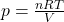Where:

n: is the number of moles of the gas

R: is the gas constant = 0.082 L*atm/(K*mol)

V: is the volume of the container = 1.64 L

T: is the temperature

We need to find the number of moles and the temperature. The number of moles is: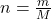Where:

M: is the molar mass of the N₂ = 14.007 g/mol*2 = 28.014 g/mol

m: is the mass of the gas = 0.226 g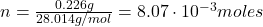Now, the temperature can be found using the following equation: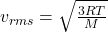Where:

R: is the gas constant = 0.082 L*atm/K*mol = 8.314 J/K*mol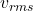: is the root-mean-square speed of the gas = 182 m/s

By solving the above equation for T, we have: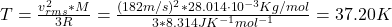Finally, we can find the pressure of the gas: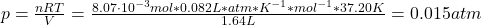Therefore, the pressure of the gas is 0.015 atm.

I hope it helps you!